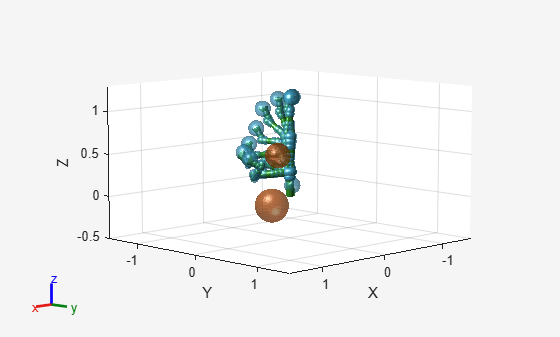# chompSolverOptions

Solver options for CHOMP motion planner

Since R2023a

## Description

The `chompSolverOptions` object stores Covariant Hamiltonian Optimization for Motion Planning (CHOMP) solver options that you can use to change the behavior of the solver.

## Creation

### Syntax

``OPTS = chompSolverOptions``
``OPTS = chompSolverOptions(Name=Value)``

### Description

````OPTS = chompSolverOptions` creates a solver options object `OPTS` that you can use to set options for a `manipulatorCHOMP` object to optimize a trajectory.```

example

````OPTS = chompSolverOptions(Name=Value)` specifies properties using one or more name-value arguments.```

## Properties

expand all

Verbosity of the diagnostic output to the command window from the `optimize` function, specified as one of these options:

• `"none"` — Output no information.

• `"concise"` — Output the objective function results.

• `"detailed"` — Output the smoothness costs, collision costs, and the objective function results.

Data Types: `char` | `string`

Termination tolerance on the objective function, specified as a nonnegative numeric scalar. The termination tolerance is the absolute relative change of the objective function between consecutive iterations:

$|\frac{{f}_{i-1}-{f}_{i}}{{f}_{i}}|$,

where f is the objective function and i is an iteration.

Maximum time to optimize the trajectory, specified as a nonnegative numeric scalar. Units are in seconds.

Maximum number of iterations to optimize the trajectory, specified as a nonnegative integer scalar.

Learning rate at which to update the objective function, specified as a positive numeric scalar. Units are in seconds.

## Examples

collapse all

Load a robot model into the workspace, and create a CHOMP solver.

```robot = loadrobot("kinovaGen3",DataFormat="row"); chomp = manipulatorCHOMP(robot);```

Create spheres to represent obstacles, and add them to the CHOMP solver.

```env = [0.20 0.2 -0.1 -0.1; % sphere, radius 0.20 at (0.2,-0.1,-0.1) 0.15 0.2 0.0 0.5]'; % sphere, radius 0.15 at (0.2,0.0,0.5) chomp.SphericalObstacles = env;```

To prioritize a collision-free trajectory, set the smoothness cost weight to a lower value than the collision cost weight. Then add the options to the CHOMP solver.

```chomp.SmoothnessOptions = chompSmoothnessOptions(SmoothnessCostWeight=1e-3); chomp.CollisionOptions = chompCollisionOptions(CollisionCostWeight=10); chomp.SolverOptions = chompSolverOptions(Verbosity="none",LearningRate=7.0);```

Initialize a trajectory, optimize it using the CHOMP solver, and show the waypoints in a figure.

```startconfig = homeConfiguration(robot); goalconfig = [0.5 1.75 -2.25 2.0 0.3 -1.65 -0.4]; timepoints = [0 5]; timestep = 0.1; trajtype = "minjerkpolytraj"; [wptsamples,tsamples] = optimize(chomp, ... [startconfig; goalconfig], ... timepoints, ... timestep, ... InitialTrajectoryFitType=trajtype); show(chomp,wptsamples,NumSamples=10); zlim([-0.5 1.3])```Ratliff, Nathan, Siddhartha Srinivasa, Matt Zucker, and Andrew Bagnell. “CHOMP: Gradient Optimization Techniques for Efficient Motion Planning.” In 2009 IEEE International Conference on Robotics and Automation, 489–94. Kobe, Japan: IEEE, 2009. https://doi.org/10.1109/ROBOT.2009.5152817.